Checkout JEE MAINS 2022 Question Paper Analysis : Checkout JEE MAINS 2022 Question Paper Analysis :

# Energy Of An Orbiting Satellite

The satellites orbit around a central massive body in either a circular or an elliptical manner. A satellite orbiting about the earth moves in a circular motion at a constant speed and at a fixed height by moving with a tangential velocity that allows it to fall at the same rate at which the earth curves. The force of gravity acts in a direction perpendicular to the direction of motion of the satellite throughout the trajectory.

As per the work-energy theorem, the initial total mechanical energy of the system plus the work done by any external force is equal to the final total mechanical energy.

Mathematically, KEi + PEi + Wext = KEf + PEf

For satellites, the force of gravity is the only external force and since gravity is considered as a conservative force, the term Wext is zero.

The equation can be simplified as KEi + PEi = KEf + PEf

In other words, the sum of the kinetic energy and the potential energy of the system is constant, while energy gets transformed between the kinetic energy and the potential energy.

## Analysis For Circular Orbits

In circular motion about the Earth, a satellite remains at a fixed distance from the surface of the Earth at all the time. Since the tangential velocity is a function of the radius of the orbit, the velocity remains constant and so does the kinetic energy. Also, since the potential energy is dependent on the height of the object, which remains constant in this case, thus, the potential energy remains constant throughout. So, the total mechanical energy i.e. KE + PE remains constant.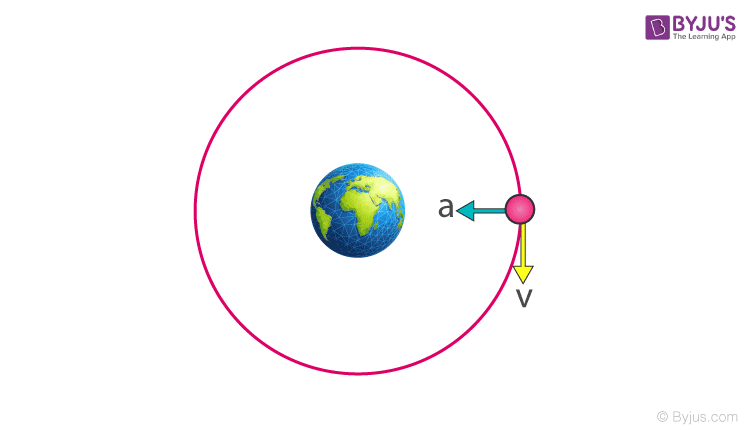## Analysis For Elliptical Orbits

The total mechanical energy of a satellite in elliptical motion also remains constant, like the case of circular motion but unlike the circular motion, the energy of a satellite in elliptical motion changes forms. As we know the tangential velocity of a body orbiting around the Earth varies inversely with the square root of the radius of its orbit, the kinetic energy also decreases with the increase in the radius and is inversely proportional to the radius of the orbit. Similarly, the potential energy increases with the increase in the height of the object and thus it increases with the increase in the radius of the orbit.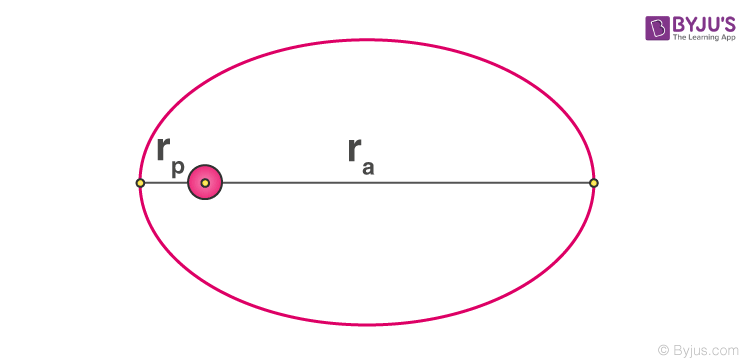## The Energy of A Circularly Orbiting Satellite

The motion of a satellite around the Earth is considered to be circular. In this section, we will derive the expression for the kinetic energy, potential energy, and the total mechanical energy of an object orbiting in a circular path around the Earth.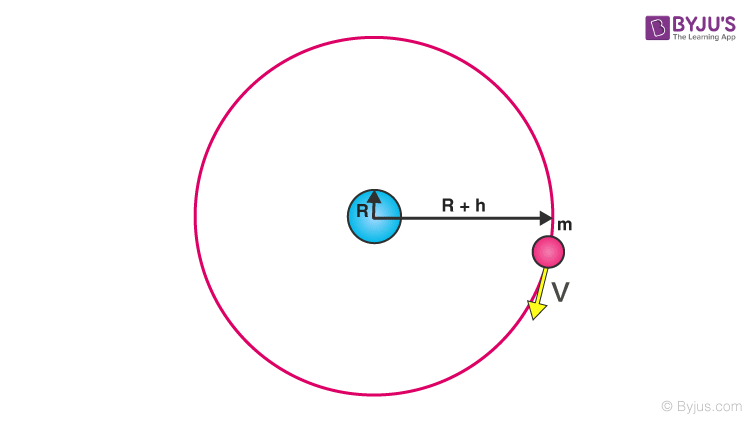For a satellite orbiting the earth, the tangential velocity can be given as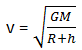Where M is the mass of the earth, R is the radius of the earth, h is the height from the surface of the earth where an object is kept.

So, the kinetic energy of the satellite (mass m) in a circular orbit with speed v can be written as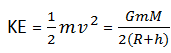As per our assumption, the gravitational potential energy at infinity is considered to be zero, so, the potential energy at distance (R +h) from the center of the earth can be written as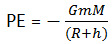The kinetic energy here is positive whereas the potential energy is negative. However, in magnitude, the kinetic energy is half the potential energy, so the total energy E is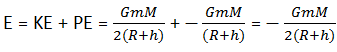The total energy of a circularly orbiting satellite is thus negative, with the potential energy being negative but twice is the magnitude of the positive kinetic energy.## Frequently Asked Questions – FAQs

### What is an artificial satellite?

An artificial satellite is a specially designed body (with special purposes) that has been placed into orbit using rockets or other propulsion systems. These bodies are called artificial satellites to differentiate them from natural celestial bodies that revolve around planets such as the Earth’s Moon.

### Which was the first artificial satellite?

An artificial satellite is a specially designed
Sputnik 1 was the first satellite.

### Explain the work-energy theorem.

According to the work-energy theorem, the initial total mechanical energy of the system plus the work done by any external force is equal to the final total mechanical energy.

### How do satellites behave in a circular orbit?

In circular motion about the Earth, a satellite stays at a fixed distance from the surface of the Earth at all times. Since the tangential velocity is a function of the radius of the orbit, the velocity stays constant, and so does the kinetic energy.

### How do satellites behave in an elliptical orbit?

The total mechanical energy of a satellite in elliptical motion also stays constant, like the scenario of circular motion, but unlike circular motion, the energy of a satellite in elliptical motion varies.
Test Your Knowledge On Energy Orbitting Satellite!